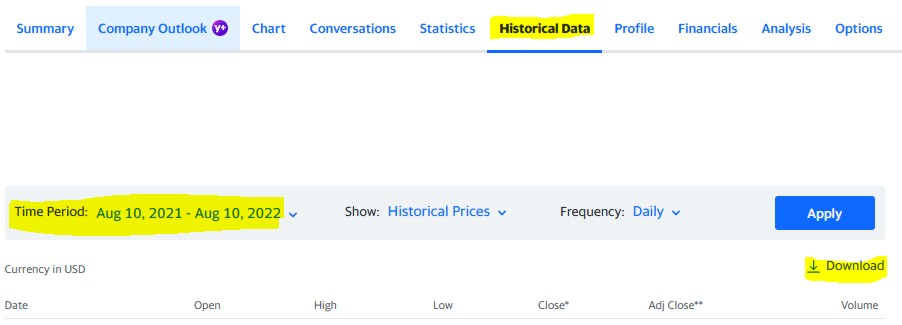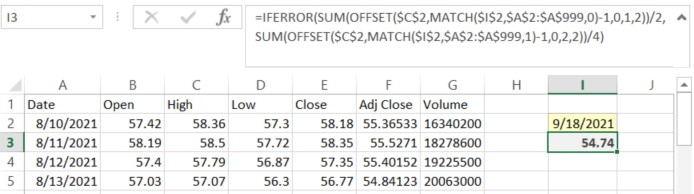# Easily Calculate the Fair Market Value (FMV) of a Security in ExcelThere are many reasons why you may need to calculate the Fair Market Value of a security on a particular day. These include the so-called step up to date of death cost basis adjustment and calculating the valuation of a charitable gift of stock for your charitable deduction.

Fortunately, the steps for calculating the fair market value of a frequently traded security are easy and straight forward. Plus, with the help of Yahoo Finance and a one-cell formula, you barely need to do any work.

First, go to Yahoo Finance at https://finance.yahoo.com/ to gather the historical pricing information.

Type the ticker symbol you are looking for into the search bar and press enter to search. On the page that loads, select “Historical Data.”Review the time period and adjust as necessary to ensure that it includes the date in question. Then, click the “Download” button. This will download a CSV file of the price data.

Currently, the CSV file that Yahoo Finance generates has the following columns in the following order A) Date, B) Open, C) High, and D) Low. Also, the data of the CSV file begins in row 2.

Assuming that your file has that format, then the following formula should locate the Fair Market Value of a weekend price. If your data is in different columns, either adjust your columns to have this organization or adjust the references to those columns in the formula.

Type the date in question in cell \$I\$2. Format it as a short date, such as 9/18/2021.

Then place in \$I\$3 the following formula:

=IFERROR(SUM(OFFSET(\$C\$2,MATCH(\$I\$2,\$A\$2:\$A\$999,0)-1,0,1,2))/2,SUM(OFFSET(\$C\$2,MATCH(\$I\$2,\$A\$2:\$A\$999,1)-1,0,2,2))/4)

This formula works in several steps.

First the “MATCH(\$I\$2,\$A\$2:\$A\$999,0)” tries to locate the precise location of the date you listed. If it finds it, it returns that location and uses it in the offset to return the array of the high price and the low price on that date. If it does not return a match, then the IFERROR identifies the error and moves into the second clause.

The second clause locates the day before the date in question and then averages the high and low prices from that row and the row directly after it. This part of the formula assumes that the data is sorted from oldest to newest (as it comes out of Yahoo by default). If you have changed the sort order, then the MATCH functions in this part of the formula will no longer work or may return the wrong answer.

If you’d like to check the work of this formula, the following formulas return various components individually, assuming that you have put the relevant date into \$I\$2:

Date Of Date Prior Date After
Date =OFFSET(\$A\$2, MATCH(\$I\$2,\$A\$2:\$A\$999,0)-1,0) =OFFSET(\$A\$2, MATCH(\$I\$2,\$A\$2:\$A\$999,1)-1,0) =OFFSET(\$A\$2, MATCH(\$I\$2,\$A\$2:\$A\$999,1),0)
High =OFFSET(\$C\$2, MATCH(\$I\$2,\$A\$2:\$A\$999,0)-1,0) =OFFSET(\$C\$2, MATCH(\$I\$2,\$A\$2:\$A\$999,1)-1,0) =OFFSET(\$C\$2, MATCH(\$I\$2,\$A\$2:\$A\$999,1),0)
Low =OFFSET(\$D\$2, MATCH(\$I\$2,\$A\$2:\$A\$999,0)-1,0) =OFFSET(\$D\$2, MATCH(\$I\$2,\$A\$2:\$A\$999,1)-1,0) =OFFSET(\$D\$2, MATCH(\$I\$2,\$A\$2:\$A\$999,1),0)

Here is the formula in action, using the example from “How to Calculate Fair Market Value if the Date of Death Is on a Weekend?Note that if you have a thinly traded security such that there are open trading days where no trading occurs, there are extra steps to calculate the fair market value. In those cases, a prorated formula according to IRS Regulations may be required. However, for frequently traded securities, I hope this Excel formula assists you.

Photo by Cup of Couple from Pexels